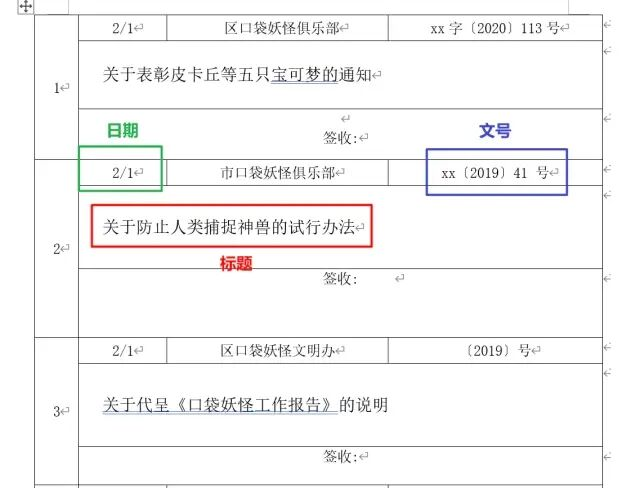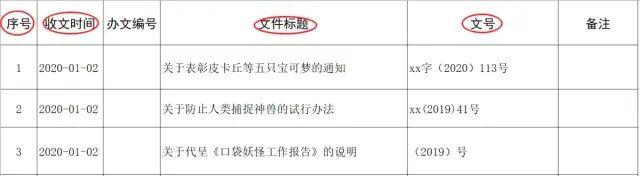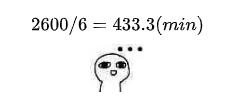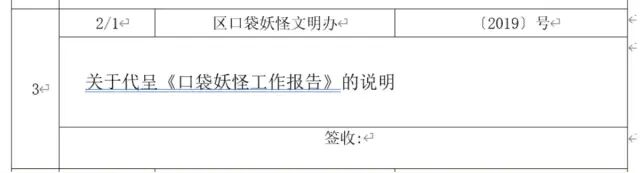#Python办公自动化之从Word到Excel

0
0
01. 云栖社区>
2. 云栖号资讯>
3. 博客>
4. 正文

## Python办公自动化之从Word到Excel# 导入需要的库docx
from docx import Document

# 指定文件存放的路径
path = r'C:\Users\word.docx'

# 读取文件
document = Document(path)

# 读取word中的所有表格
tables = document.tables 

# 获取第一张表
table0 = tables# 在全局放一个变量用来计数填序号
n = 0
for i in range(0, len(table0.rows) + 1, 3):
# 日期
date = table0.cell(i, 1).text
# 标题
title = table0.cell(i + 1, 1).text.strip()
# 文号
dfn = tables[j].cell(i, 3).text.strip()
print(n, date, tite, dfn) 

strptime: 解析字符串中蕴含的时间
strftime: 转化成所需的时间格式

import datetime

n = 0
for i in range(0, len(table0.rows) + 1, 3):
# 日期
date = table0.cell(i, 1).text
# 有的条目时间是空的，这里不做过多判别
if '/' in date:
date = datetime.datetime.strptime(date, '%d/%m').strftime('2020-%m-%d')
else:
date = '-'
# 标题
title = table0.cell(i + 1, 1).text.strip()
# 文号
dfn = tables[j].cell(i, 3).text.strip()
print(n, date, tite, dfn) 

n = 0
for j in range(len(tables)):
for i in range(0, len(tables[j].rows)+1, 3):
try:
# 日期
date = tables[j].cell(i, 1).text
if '/' in date:
date = datetime.datetime.strptime(date, '%d/%m').strftime('2020-%m-%d')
else:
date = '-'
# 标题
title = tables[j].cell(i + 1, 1).text.strip()
# 文号
dfn = tables[j].cell(i, 3).text.strip()
n += 1
print(n, date, title, dfn)
except Exception as error:
# 捕获异常，也可以用log写到日志里方便查看和管理
print(error)
continue 

from openpyxl import Workbook

# 实例化
wb = Workbook()
# 获取当前sheet
sheet = wb.active
# 设立表头
header = ['序号', '收文时间', '办文编号', '文件标题', '文号', '备注']
sheet.append(header) 

row = [n, date, ' ', title, dfn, ' ']
sheet.append(row) 

wb.save(r'C:\Users\20200420.xlsx') 

from docx import Document
import datetime
from openpyxl import Workbook

wb = Workbook()
sheet = wb.active
header = ['序号', '收文时间', '办文编号', '文件标题', '文号', '备注']

path = r'C:\Users\word.docx'
document = Document(path)
tables = document.tables

n = 0
for j in range(len(tables)):
for i in range(0, len(tables[j].rows)+1, 3):
try:
# 日期
date = tables[j].cell(i, 1).text
if '/' in date:
date = datetime.datetime.strptime(date, '%d/%m').strftime('2020-%m-%d')
else:
date = '-'
# 标题
title = tables[j].cell(i + 1, 1).text.strip()
# 文号
dfn = tables[j].cell(i, 3).text.strip()
n += 1
print(n, date, title, dfn)
row = [n, date, ' ', title, dfn, ' ']
sheet.append(row)
except Exception as error:
# 捕获异常，也可以用log写到日志里方便查看和管理
print(error)
continue

wb.save(r'C:\Users\20200420.xlsx') 

【云栖号在线课堂】每天都有产品技术专家分享！

【云栖号在线课堂 社群】https://c.tb.cn/F3.Z8gvnK

+ 关注